# Consider the Lewis structure of IBr4, what is the bond angle between Br-l-Br? © < 109.5°...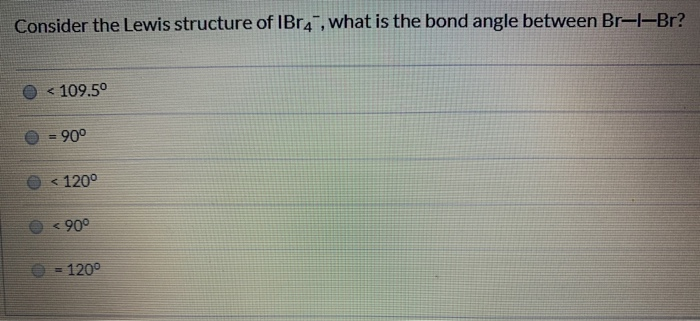Consider the Lewis structure of IBr4, what is the bond angle between Br-l-Br? © < 109.5° O = 90° 0 <1200 < 90° 0 = 120°

Bond angle between Br-I-Br is 90°.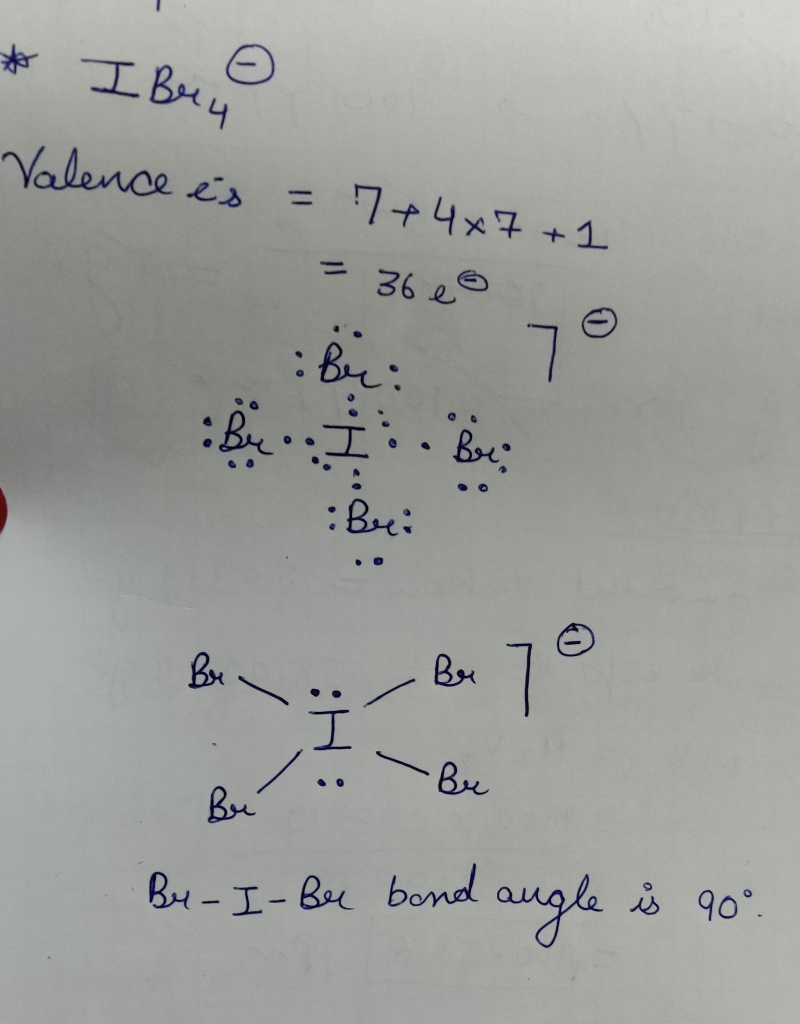#### Earn Coin

Coins can be redeemed for fabulous gifts.

Similar Homework Help Questions
• ### Question 10 1 pts Consider the Lewis structure of Br4 , what is the bond angle...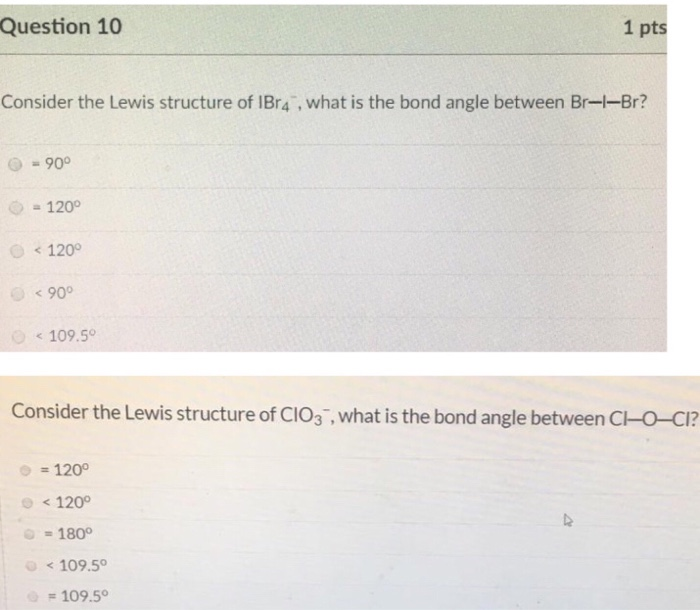Question 10 1 pts Consider the Lewis structure of Br4 , what is the bond angle between Br-1-Br? = 90° = 120° < 120° < 90° < 109.50 Consider the Lewis structure of CIO3 , what is the bond angle between C-O-CI? = 120° < 120° = 180° < 109.50 = 109.50

• ### Question 10 1 pts Consider the Lewis structure of IF 2 , what is the bond...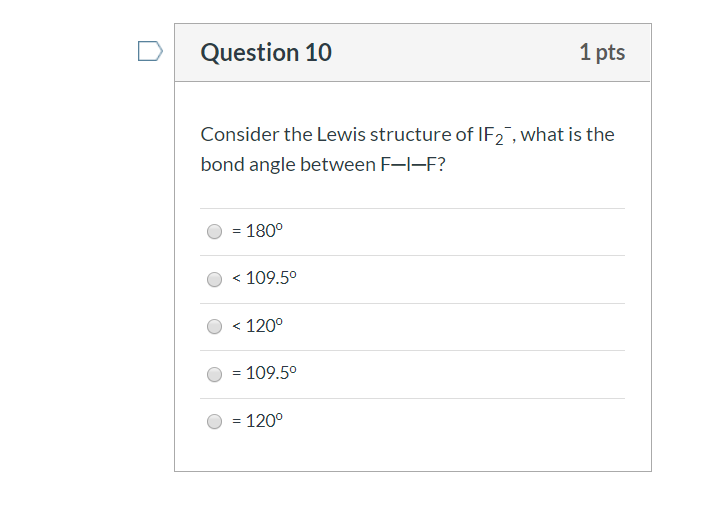Question 10 1 pts Consider the Lewis structure of IF 2 , what is the bond angle between F-I-F? = 180° < 109.5° O < 120° = 109.50 O = 120°

• ### Question 10 1 pts Consider the Lewis structure of IF7, what is the bond angle between...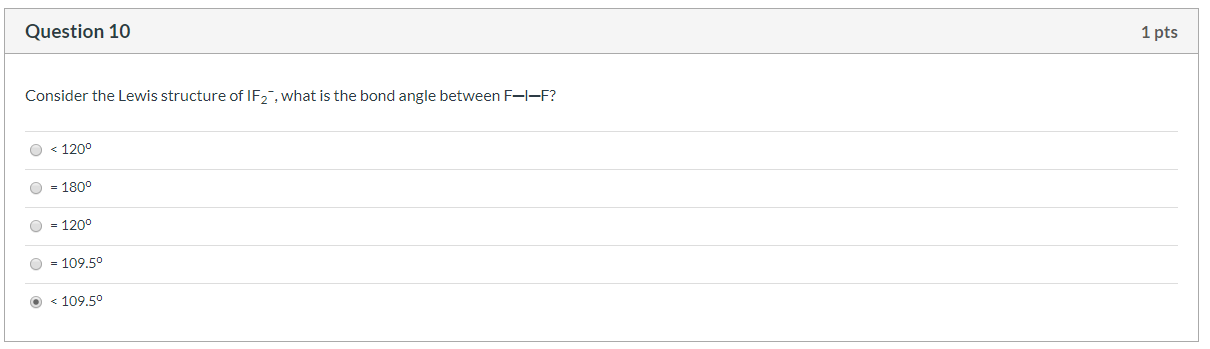Question 10 1 pts Consider the Lewis structure of IF7, what is the bond angle between F-I-F? <1200 1800 1200 109.50 <109.50

• ### Select the correct value for the indicated bond angle in each of the compounds. 0-S-O angle...Select the correct value for the indicated bond angle in each of the compounds. 0-S-O angle of S02 F-B-F angle of BF3 O 120° 0 <120° 0 <109.5° O 109.5° 0 <120° O 120° 0 <109.5° O 90° O 180° O 109.5° O 90° O 180° F-0-F angle of OF 2 Cl-Be-Cl angle of BeCl2 O <109.5° 0 <120° O 90° O 120° O 180° O 109.5° O 180° O 90° O <109.50 O 109.5° O 120° 0 <120° F-P-F...

• ### Question 5 (3 points) What is the bond angle between the two O-H bonds in H20?!...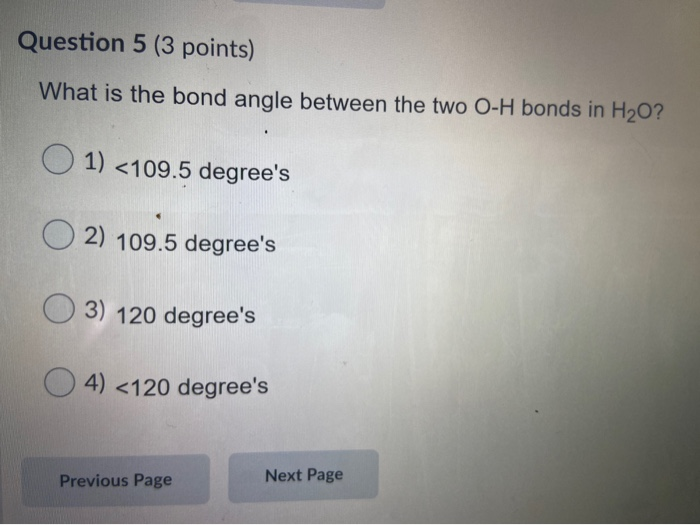Question 5 (3 points) What is the bond angle between the two O-H bonds in H20?! O 1) <109.5 degree's O2) 109.5 degree's 03) 120 degree's 4) <120 degree's Previous Page Next Page

• ### Question 2 2.5 pts A molecule with a seesaw molecular geometry has a bond angle of...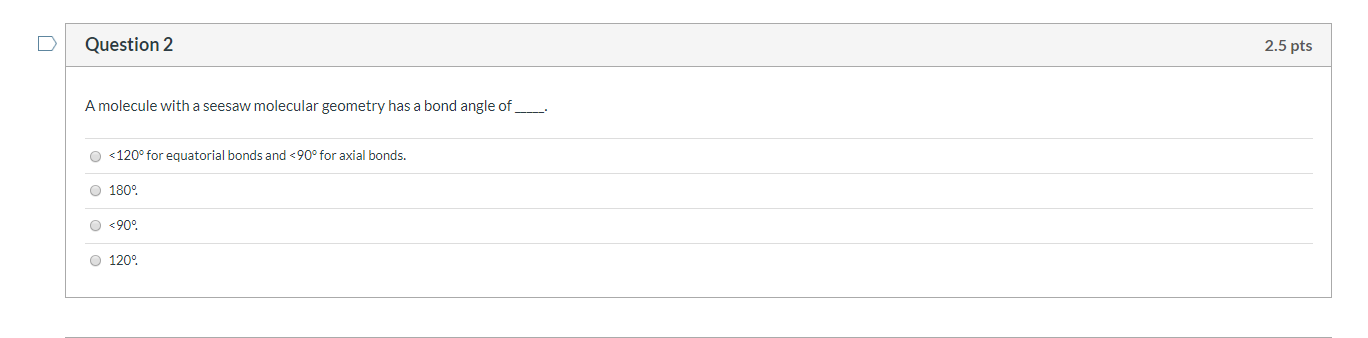Question 2 2.5 pts A molecule with a seesaw molecular geometry has a bond angle of __ <120° for equatorial bonds and <90° for axial bonds. 180° <909 1200

• ### Determine if each of the following statements is True or False about sigma (o) bonds and...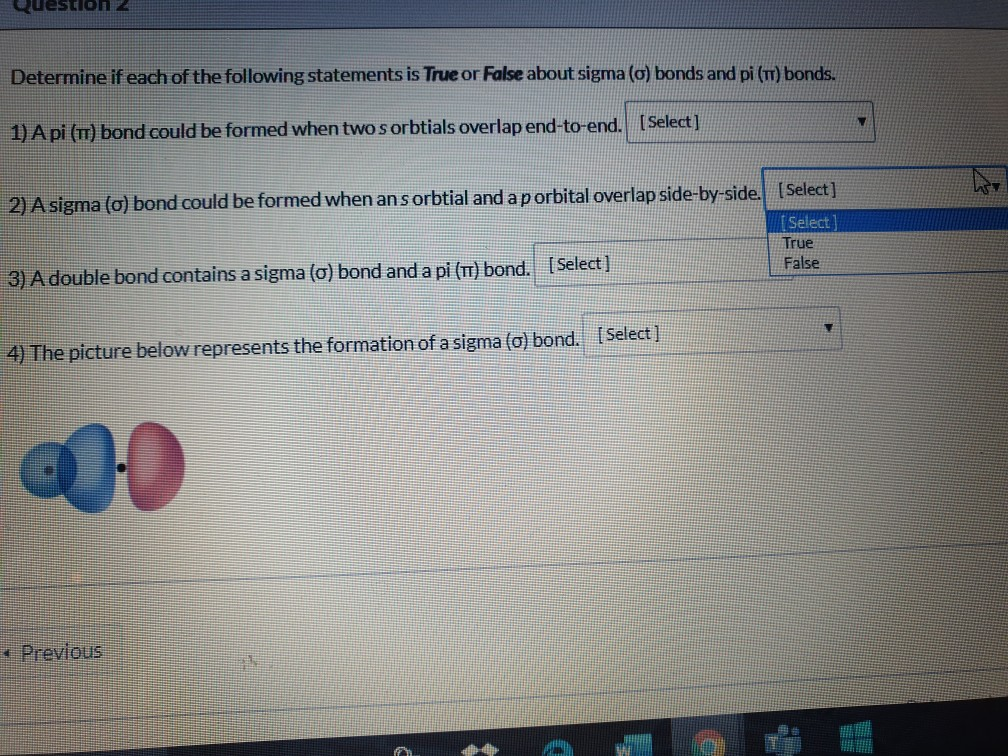Determine if each of the following statements is True or False about sigma (o) bonds and pi (m) bonds. [Select] 1) A pi (T) bond could be formed when twosorbtials overlap end-to-end. 2) A sigma (o) bond could be formed when an s orbtial and a p orbital overlap side-by-side. [Select] [Select] True False [Select ] 3) A double bond contains a sigma (o) bond and a pi (TT) bond. [Select) 4) The picture below represents the formation of a...

• ### Draw Lewis Structure • Predict geometry • Predict bond angle • Predict podarity > SiO2 >...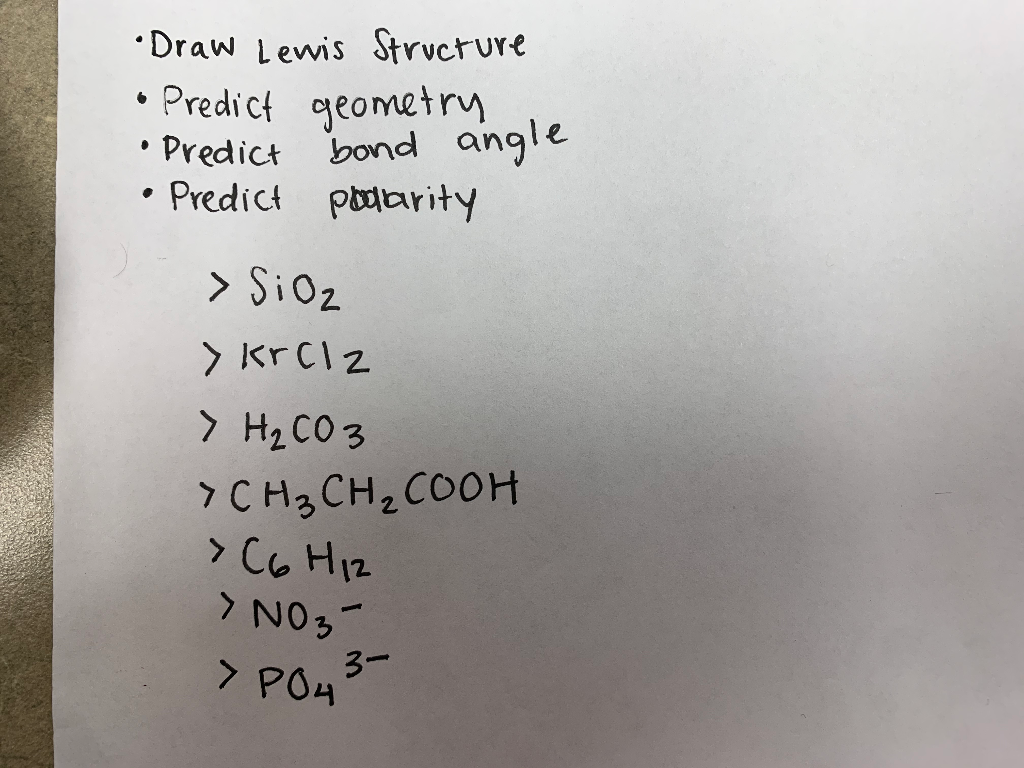Draw Lewis Structure • Predict geometry • Predict bond angle • Predict podarity > SiO2 > Krclz > H₂CO3 > CH3CH2COOH C6H12 NO₃- > PO43-

• ### Answer this question about the structure you’ve drawn for SeCl2. What is the bond angle? 180°...

Answer this question about the structure you’ve drawn for SeCl2. What is the bond angle? 180° 120° <109.5° <120° Can’t determine the bond angle based on the Lewis structure. 109.5°

• ### Question 8 What is the approximate bond angle around Silicon in SiF4? Choose the best response....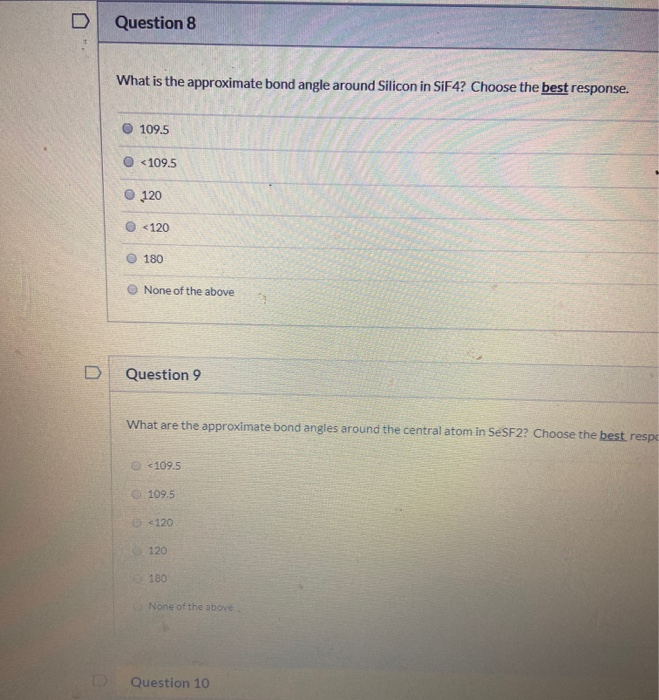Question 8 What is the approximate bond angle around Silicon in SiF4? Choose the best response. 109.5 <109.5 120 <120 180 None of the above Question 9 What are the approximate bond angles around the central atom in SeSF22 Choose the best respo <109.5 109.5 6 <120 120 180 None of the above Question 10